# Problem of the Day March 26, 2020

Mar 26, 2020Problem of the Day March 26, 2020

Label the three triangles as right, obtuse or acute and equilateral, isoceles, or scalene. Find the missing side length in triangle ABC.Find the area and the circumference of the circles below:Plot the following points on the coordinate plane: ( 4 , 2 ) , ( -2 , 4 ) and

( -6 , -4)

Algebra

Write the equation of the line through the points ( - 2, 4 ) and ( 6, 8 ).

Geometry

Find the measure of the labeled angles. Name the Theorem you used to get your answer. (Alternate Interior Angles, Consecutive Angles, Corresponding Angles, Vertical Angles, Alternate Exterior Angles)Algebra 2

Graph the following system of inequalities:

-3x + y < 2  and 2x + y > 1

Trigonometry

Use sin, cos and tan to find the labeled sides or angles.Pre-Calculus

Graph the following sin function: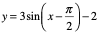Grade 6: Triangle ABC right scalene missing side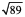Triangle GHI acute equilateral

Triangle PQR scalene obtuse

Grade 7: area of circle A 36pi circumference 12pi

area of circle  C 9pi  circumference 6pi

Algebra: y = 1/2x + 5

Geometry: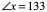consecutive angles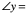47  corresponding angles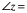133 alternate exterior angles or consecutive angles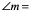47  alternate exterior angles or vertical angles

Algebra 2:Trigonometry: tan 30 = 4/x   6.928

tan 50 = 7/y  5.874

Pre-Calculus: